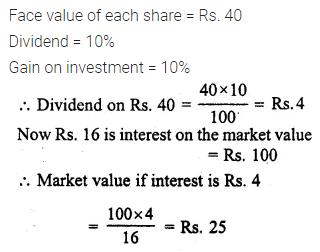# ML Aggarwal Class 10 Solutions for ICSE Maths Chapter 3 Shares and Dividends Chapter Test

## ML Aggarwal Class 10 Solutions for ICSE Maths Chapter 3 Shares and Dividends Chapter Test

ML Aggarwal Class 10 Solutions for ICSE Maths Chapter 3 Shares and Dividends Chapter Test

Question 1.
If a man received ₹1080 as dividend from 9% ₹20 shares, find the number of shares purchased by him.
Solution: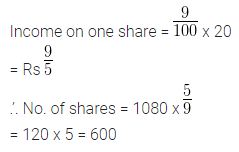Question 2.
Find the percentage interest on capital invested in 18% shares when a Rs 10 share costs Rs 12.
Solution:Question 3.
Rohit Kulkarni invests Rs 10000 in 10% Rs 100 shares of a company. If his annual dividend is Rs 800, find :
(i) The market value of each share.
(ii) The rate per cent which he earns on his investment.
Solution:Question 4.
At what price should a 9% Rs 100 share be quoted when the money is worth 6%?
Solution: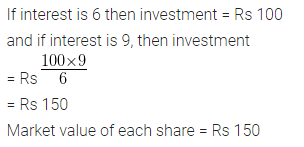Question 5.
By selling at Rs 92, some 2.5% Rs 100 shares and investing the proceeds in 5% Rs 100 shares at Rs 115, a person increased his annual income by Rs 90. Find:
(i) the number of shares sold.
(ii) the number of shares purchased.
(iii) the new income.
(iv) the rate per cent which he earns on his investment.
Solution: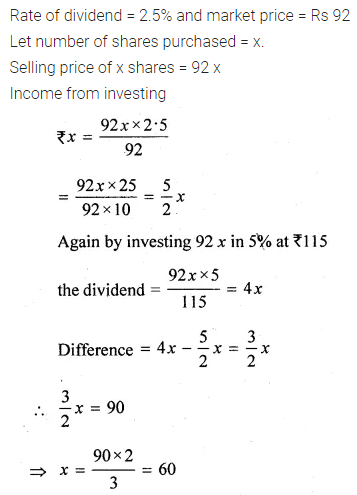Question 6.
A man has some shares of Rs. 100 par value paying a 6% dividend. He sells half of these at a discount of 10% and invests the proceeds in 7% Rs. 50 shares at a premium of Rs. 10. This transaction decreases his income from dividends by Rs. 120. Calculate:
(i) the number of shares before the transaction.
(ii) the number of shares he sold.
(iii) his initial annual income from shares.
Solution: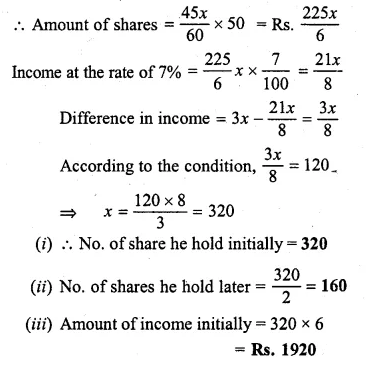Question 7.
Divide Rs. 101520 into two parts such that if one part is invested in 8% Rs. 100 shares at 8% discount and the other in 9% Rs. 50 shares at 8% premium, the annual incomes are equal.
Solution: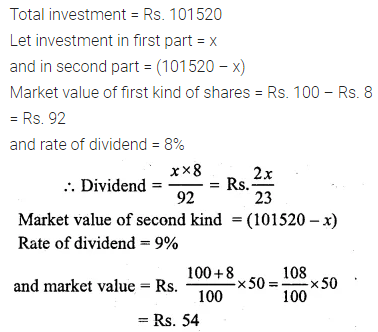Question 8.
A man buys Rs. 40 shares of a company which pays a 10% dividend. He buys the shares at such a price that his profit is 16% on his investment. At what price did he buy each share?
Solution: